2016“福特汽车环保奖”全面启动

【一猫汽车网西安站】2016年6月13日，四川成都——2016“福特汽车环保奖”启动仪式在天府之国成都举行。今年，福特汽车环保奖将以“保护生物多样性，共建生态文明”为主题，围绕生物多样性保护展开一系列环保宣传教育行动。在启动仪式上，与会嘉宾通过参与完成绘有中国代表性动植物的画作，正式揭开了2016“福特汽车环保奖”的序幕。并对王朗国家级自然保护区展开了为期两天的获奖项目回访活动。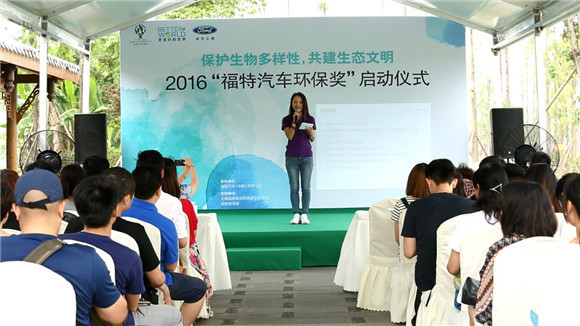“福特汽车环保奖”是由福特汽车发起的全球性环保奖项评选活动，是福特“更美好的世界”公益项目的重要组成部分。2000年，“福特汽车环保奖”首次进入中国，十六年来，“福特汽车环保奖”在中国累计资助382个优秀环保团体和个人，授予奖金2,010万人民币。2016年，“福特汽车环保奖”奖金总额为人民币200万元，设有“自然环境保护—先锋奖”、“自然环境保护—传播奖”、“社区实践奖”三类奖项，采用线上申请方式，从6月13日到8月15日接受环保项目申请。值得一提的是从今年开始，“福特汽车环保奖”也将与阿拉善生态基金会、南都公益基金会、合一绿学院、广东省千禾社区公益基金会等环保资助机构合作，共同探讨及完善对环保组织的支持。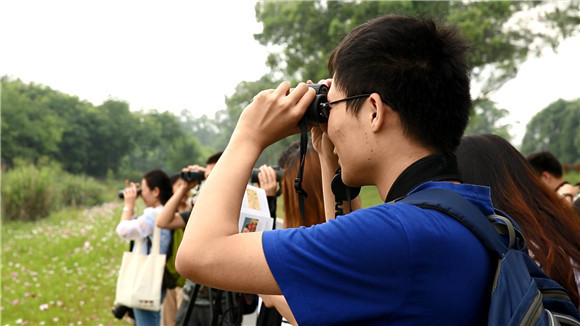上图：成都观鸟会工作人员带领回访小组观鸟

福特汽车环保奖评委杨百瑾女士，西南地区重要环保组织成都观鸟会、成都根与芽环境文化交流中心、山水自然保护中心的相关负责人与其他成都本地环保组织、环保社团和来自全国各地的20余家媒体代表参与了本次活动。启动仪式结束后，与会嘉宾还共同参与了随之举行的“公众参与西南生物多样性保护”的主题沙龙。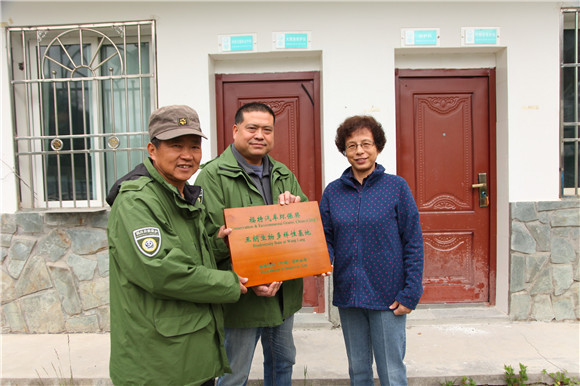上图：福特汽车环保奖评委杨百瑾女士为成都观鸟会颁发“福特汽车环保奖成都观鸟会体验基地”证书；

今年的启动仪式及获奖项目回访活动首次与国内著名环境保护组织——自然之友进行合作，全程采取“零废弃会议”的操作方式，通过会议文件电子化、活动物料尽可能回收使用、现场垃圾分类回收等方式，努力在活动过程中减少物品浪费，接近“零废弃”。最终会场共产生0.4kg垃圾，平均每人产生不足7g；会议主要物料均可反复使用，不能反复利用的会议背景布在使用后捐赠给成都爱之家动物救助中心，作为挡雨布，为小动物搭建笼舍。这也体现了福特汽车一贯秉持的环保理念以及对中国环保组织绿色行动的支持与鼓励。

环保组织共聚一堂，探讨公众参与生物多样性保护

近几年，伴随国家政策从顶层设计到全面部署，生态文明的理念已逐渐深入人心，生物多样性作为生态系统稳定的基础，其重要性也逐渐被公众所了解。开展生物多样性宣传与教育是生物多样性保护工作的重要措施，也是众多环保组织介入这一议题的主要手段。

中国西南山地是全球34个生物多样性热点地区之一，在生物多样性保护方面具有极其重要的地位。自2000年在中国启动‘福特汽车环保奖’以来，该区域内已有逾50个环保组织和个人获得过‘福特汽车环保奖’。本次启动仪式首次在四川举行，就是希望在生物多样性保护的主战场，与经验丰富的本地环保组织共同探讨西南的生物多样性保护。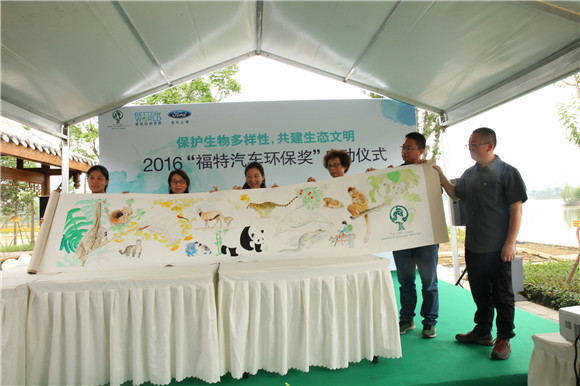上图：福特汽车（中国）有限公司亚太区企业传播总监曹鸾姿女士、成都观鸟会理事长沈尤先生、山水自然保护中心社区保护地项目主任冯杰先生、成都根与芽环境文化交流中心执行主任罗丹女士展示生物多样性画卷；
在以“公众参与西南生物多样性保护”为主题的沙龙上，成都观鸟会理事长沈尤先生、山水自然保护中心社区保护地项目主任冯杰先生、成都根与芽环境文化交流中心执行主任罗丹女士做了主题发言。三家获奖组织均在公众参与方面有多年的实践和探索，沙龙上阐述了各自在城市环境教育、城市周边生态旅游，以及保护区社区生计等方面的工作，展示出公众在参与生物多样性保护方面的丰富渠道和积极角色，并就如何更好地调动公众参与积极性等问题展开讨论。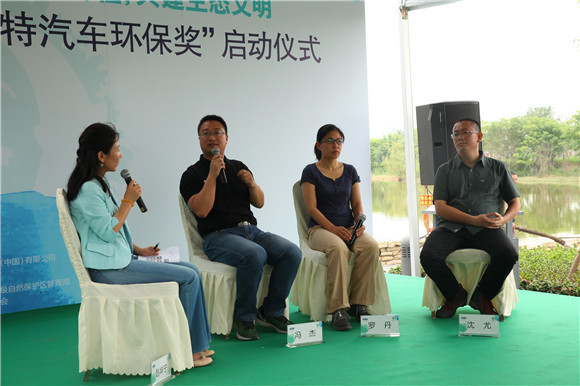上图：嘉宾参与“公众参与西南生物多样性保护”主题沙龙

探寻大熊猫踪迹，见证保护区多层次的生物多样性保护

启动仪式后，由福特汽车代表，福特汽车环保奖评委专家及10名媒体代表组成的项目回访小组驱车前往王朗国家级自然保护区。多年来，王朗国家级自然保护区致力于生物多样性监测、大熊猫栖息地保护、森林生态系统监测工作，以及生态旅游及自然教育的培育等。其“监测巡护和反偷猎项目”、“森林多重效益和碳汇项目”分别于2004年和2008年获得福特汽车环保奖“自然环境保护”二等奖。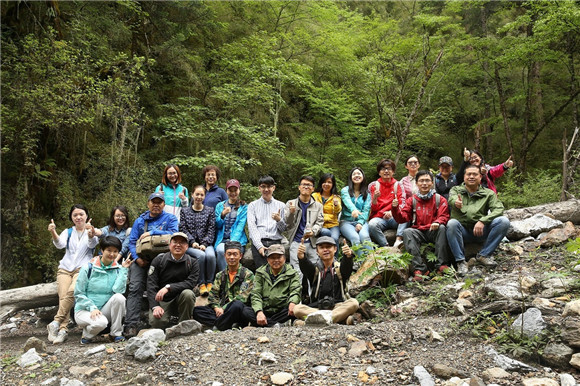上图： 回访小组合影；

王朗国家级自然保护区管理局局长蒋仕伟带领回访小组走进了大熊猫栖息地，寻找大熊猫足迹并详细介绍了保护区如何利用“福特汽车环保奖”奖金完善大熊猫监测和巡护体系、更新野外设备。谈及获得“福特汽车环保奖”对保护区的意义，蒋局长表示“除了资金的支持，两次获奖更重要的是对我们保护工作的高度肯定。这份荣誉使我们得到了更多来自外界的支持，极大地提升了保护区工作人员基层环保的热情和信心。生物多样性保护需要全社会的共同努力，王朗自然保护区非常愿意为这项事业贡献出自己的力量。”

据了解，保护区的大样地的森林监测体系已被纳入了史密森学会全球地球观测（SIGEO）网络。该网络在全球的植被群落结构、生物多样性、碳循环等方面的长期变化趋势监测中处于核心及领军位置。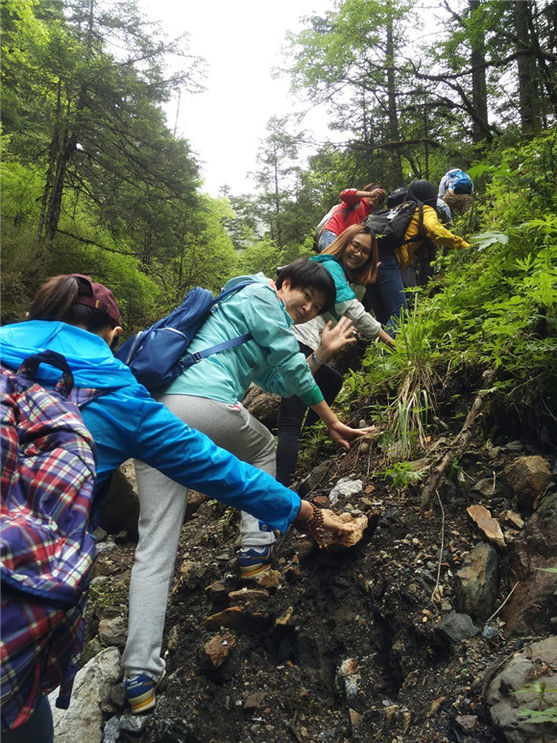上图： 回访小组探寻熊猫痕迹

生态旅游与自然知识讲座是保护区特色的公众参与手段。回访下组一行人随讲解人员前往竹根岔景区，体验生态旅游线路，了解保护区特色的森林生态系统以及在开展生态旅游过程中为保护生态环境做出的努力。在聆听过保护区自然知识讲座后，此次回访活动圆满结束。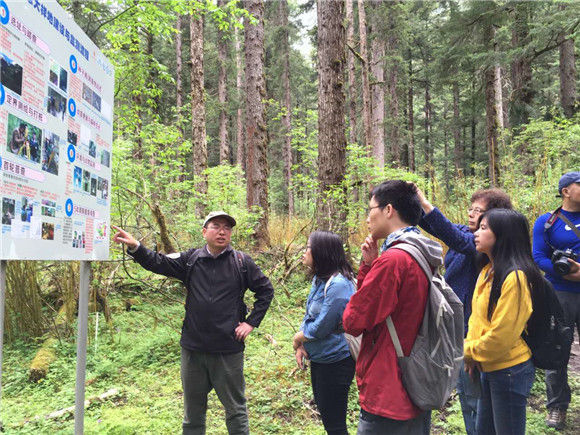上图：王朗国家级自然保护区工作人员讲解森林生态

关于福特“更美好的世界”公益项目

福特“更美好的世界”是福特汽车公司为实现企业、环境和社区可持续发展而发起的战略性企业社会责任项目。福特汽车公司相信，一个伟大的公司除了为顾客提供优质的产品和服务，还竭尽全力使世界更加美好。在中国，福特汽车将环境保护作为回报所在社区、建设更美好的世界的一个重要组成部分，并受此感召于2000年正式启动“福特汽车环保奖”，即国内最早由企业发起和组织的支持民间环保力量的奖项评选活动。2012年福特中国继续扩大对中国民间环保力量的投入，在“福特汽车环保奖”之外，推出了针对民间环保组织的能力建设项目“Level Up!绿色晋级”计划以及“福特员工志愿行动”，并整合成为福特“更美好的世界”。迄今，项目已累计资助382家优秀环保团体和个人，授予奖金2010万，为520余家民间环保组织提供能力建设培训、辅导或孵化，动员逾10000名员工及其家人参与志愿服务，累计服务时长近50000小时。
买车上一猫！
编辑  晓平

##### 相关资讯

|分享

•已阅！握爪
•我手滑为你点赞
•128个赞！
•不明觉厉
•阅后既醉
•有钱！任性
•照片太美，我不敢看
•干货！杠杠的
•高大上
•膜拜中！
•请接受我的膝盖
•猫哥/猫妹么么哒
•神吐槽
•我只笑笑不说话
•我想静静
•窒息
##### 资讯相关车系##### 热度排行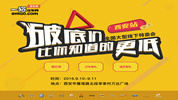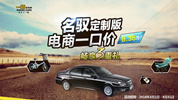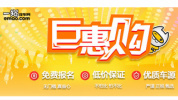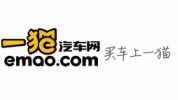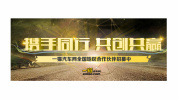• 阿斯顿·马丁
• 奥迪
• 阿尔法罗密欧
• ALPINA

• 巴博斯
• 宝骏
• 宝马
• 保时捷
• 北汽制造
• 奔驰
• 奔腾
• 本田
• 比亚迪
• 标致
• 别克
• 宾利
• 布加迪
• 北汽威旺
• 北京
• 北汽绅宝
• 北汽幻速
• 北汽新能源
• 宝沃
• 比速汽车
• 北汽道达

• 昌河
• 长安
• 长城
• 长安商用
• 成功汽车
• 长江EV
• 长安轻车型

• 大众
• 道奇
• 东风
• 东风风神
• 东风小康
• 东南
• DS
• 东风风行
• 东风风度
• 东风风光

• 法拉利
• 菲亚特
• 丰田
• 福迪
• 福特
• 福田汽车
• 福汽启腾
• 风诺

• GMC
• 广汽传祺
• 广汽吉奥
• 观致

• 哈飞
• 海格
• 海马
• 华泰
• 黄海
• 恒天
• 红旗
• 哈弗
• 华颂
• 华凯
• 华泰新能源
• 汉腾汽车

• Jeep
• 江淮
• 江铃
• 捷豹
• 金杯
• 九龙
• 吉利汽车
• 金旅
• 金龙
• 江铃集团轻汽
• 江铃集团新能源
• 君马汽车
• 捷途

• 开瑞
• 凯迪拉克
• 科尼赛克
• 克莱斯勒
• KTM
• 卡威
• 凯翼
• 康迪
• 康迪电动汽车集团

• 猎豹汽车
• 兰博基尼
• 劳斯莱斯
• 雷克萨斯
• 雷诺
• 理念
• 力帆
• 莲花汽车
• 林肯
• 铃木
• 陆风
• 路虎
• 路特斯
• 领志
• 领克
• 零跑汽车

• MG
• MINI
• 马自达
• 玛莎拉蒂
• 迈凯伦
• 摩根

• 纳智捷

• 讴歌
• 欧宝
• 欧朗
• 欧拉
• 欧尚汽车

• 帕加尼

• 奇瑞
• 启辰
• 起亚
• 前途
• 庆铃汽车

• 日产
• 荣威
• 瑞麒汽车
• 如虎
• 瑞驰

• 上汽大通
• smart
• 三菱
• 双环
• 双龙
• 斯巴鲁
• 斯柯达
• 萨博
• 思铭
• 赛麟
• SWM斯威汽车

• TESLA
• 泰卡特
• 腾势

• 威麟
• 威兹曼
• 沃尔沃
• 五菱汽车
• 五十铃
• 潍柴英致
• WEY
• 蔚来
• 威马汽车

• 现代
• 雪佛兰
• 雪铁龙
• 西雅特
• 新特汽车
• 小鹏汽车
• 新宝骏

• 野马汽车
• 一汽
• 依维柯
• 英菲尼迪
• 永源
• 驭胜

• 中华
• 中兴
• 众泰
• 知豆
• 之诺
• 正道汽车
• A
• B
• C
• D
• E
• F
• G
• H
• I
• J
• K
• L
• M
• N
• O
• P
• Q
• R
• S
• T
• U
• V
• W
• X
• Y
• Z

• 阿斯顿·马丁
• 奥迪
• 阿尔法罗密欧
• ALPINA

• 巴博斯
• 宝骏
• 宝马
• 保时捷
• 北汽制造
• 奔驰
• 奔腾
• 本田
• 比亚迪
• 标致
• 别克
• 宾利
• 布加迪
• 北汽威旺
• 北京
• 北汽绅宝
• 北汽幻速
• 北汽新能源
• 宝沃
• 比速汽车
• 北汽道达

• 昌河
• 长安
• 长城
• 长安商用
• 成功汽车
• 长江EV
• 长安轻车型

• 大众
• 道奇
• 东风
• 东风风神
• 东风小康
• 东南
• DS
• 东风风行
• 东风风度
• 东风风光

• 法拉利
• 菲亚特
• 丰田
• 福迪
• 福特
• 福田汽车
• 福汽启腾
• 风诺

• GMC
• 广汽传祺
• 广汽吉奥
• 观致

• 哈飞
• 海格
• 海马
• 华泰
• 黄海
• 恒天
• 红旗
• 哈弗
• 华颂
• 华凯
• 华泰新能源
• 汉腾汽车

• Jeep
• 江淮
• 江铃
• 捷豹
• 金杯
• 九龙
• 吉利汽车
• 金旅
• 金龙
• 江铃集团轻汽
• 江铃集团新能源
• 君马汽车
• 捷途

• 开瑞
• 凯迪拉克
• 科尼赛克
• 克莱斯勒
• KTM
• 卡威
• 凯翼
• 康迪
• 康迪电动汽车集团

• 猎豹汽车
• 兰博基尼
• 劳斯莱斯
• 雷克萨斯
• 雷诺
• 理念
• 力帆
• 莲花汽车
• 林肯
• 铃木
• 陆风
• 路虎
• 路特斯
• 领志
• 领克
• 零跑汽车

• MG
• MINI
• 马自达
• 玛莎拉蒂
• 迈凯伦
• 摩根

• 纳智捷

• 讴歌
• 欧宝
• 欧朗
• 欧拉
• 欧尚汽车

• 帕加尼

• 奇瑞
• 启辰
• 起亚
• 前途
• 庆铃汽车

• 日产
• 荣威
• 瑞麒汽车
• 如虎
• 瑞驰

• 上汽大通
• smart
• 三菱
• 双环
• 双龙
• 斯巴鲁
• 斯柯达
• 萨博
• 思铭
• 赛麟
• SWM斯威汽车

• TESLA
• 泰卡特
• 腾势

• 威麟
• 威兹曼
• 沃尔沃
• 五菱汽车
• 五十铃
• 潍柴英致
• WEY
• 蔚来
• 威马汽车

• 现代
• 雪佛兰
• 雪铁龙
• 西雅特
• 新特汽车
• 小鹏汽车
• 新宝骏

• 野马汽车
• 一汽
• 依维柯
• 英菲尼迪
• 永源
• 驭胜

• 中华
• 中兴
• 众泰
• 知豆
• 之诺
• 正道汽车
• A
• B
• C
• D
• E
• F
• G
• H
• I
• J
• K
• L
• M
• N
• O
• P
• Q
• R
• S
• T
• U
• V
• W
• X
• Y
• Z

• 阿斯顿·马丁
• 奥迪
• 阿尔法罗密欧
• ALPINA

• 巴博斯
• 宝骏
• 宝马
• 保时捷
• 北汽制造
• 奔驰
• 奔腾
• 本田
• 比亚迪
• 标致
• 别克
• 宾利
• 布加迪
• 北汽威旺
• 北京
• 北汽绅宝
• 北汽幻速
• 北汽新能源
• 宝沃
• 比速汽车
• 北汽道达

• 昌河
• 长安
• 长城
• 长安商用
• 成功汽车
• 长江EV
• 长安轻车型

• 大众
• 道奇
• 东风
• 东风风神
• 东风小康
• 东南
• DS
• 东风风行
• 东风风度
• 东风风光

• 法拉利
• 菲亚特
• 丰田
• 福迪
• 福特
• 福田汽车
• 福汽启腾
• 风诺

• GMC
• 广汽传祺
• 广汽吉奥
• 观致

• 哈飞
• 海格
• 海马
• 华泰
• 黄海
• 恒天
• 红旗
• 哈弗
• 华颂
• 华凯
• 华泰新能源
• 汉腾汽车

• Jeep
• 江淮
• 江铃
• 捷豹
• 金杯
• 九龙
• 吉利汽车
• 金旅
• 金龙
• 江铃集团轻汽
• 江铃集团新能源
• 君马汽车
• 捷途

• 开瑞
• 凯迪拉克
• 科尼赛克
• 克莱斯勒
• KTM
• 卡威
• 凯翼
• 康迪
• 康迪电动汽车集团

• 猎豹汽车
• 兰博基尼
• 劳斯莱斯
• 雷克萨斯
• 雷诺
• 理念
• 力帆
• 莲花汽车
• 林肯
• 铃木
• 陆风
• 路虎
• 路特斯
• 领志
• 领克
• 零跑汽车

• MG
• MINI
• 马自达
• 玛莎拉蒂
• 迈凯伦
• 摩根

• 纳智捷

• 讴歌
• 欧宝
• 欧朗
• 欧拉
• 欧尚汽车

• 帕加尼

• 奇瑞
• 启辰
• 起亚
• 前途
• 庆铃汽车

• 日产
• 荣威
• 瑞麒汽车
• 如虎
• 瑞驰

• 上汽大通
• smart
• 三菱
• 双环
• 双龙
• 斯巴鲁
• 斯柯达
• 萨博
• 思铭
• 赛麟
• SWM斯威汽车

• TESLA
• 泰卡特
• 腾势

• 威麟
• 威兹曼
• 沃尔沃
• 五菱汽车
• 五十铃
• 潍柴英致
• WEY
• 蔚来
• 威马汽车

• 现代
• 雪佛兰
• 雪铁龙
• 西雅特
• 新特汽车
• 小鹏汽车
• 新宝骏

• 野马汽车
• 一汽
• 依维柯
• 英菲尼迪
• 永源
• 驭胜

• 中华
• 中兴
• 众泰
• 知豆
• 之诺
• 正道汽车
• A
• B
• C
• D
• E
• F
• G
• H
• I
• J
• K
• L
• M
• N
• O
• P
• Q
• R
• S
• T
• U
• V
• W
• X
• Y
• Z